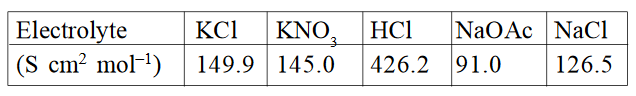####Calculate molar conductance of acetic acid using appropriate molar conductances of the electrolytes listed above at infinite dilution inat 25°C. Option 1)Option 2)Option 3)Option 4)As we learnt in

Formula for limiting molar conductivity for electrolyte -are limiting molar conductivities of cation and anion respectively.

- wherein

if an electrolyte on dissociation givesanions.Option 1)This option is incorrect.

Option 2)This option is incorrect.

Option 3)This option is correct.

Option 4)This option is incorrect.

#### Vakul